# Abstracts

The five parameter gamma-Weibull distribution has been introduced by Leipnik and Pearce (2004). Nadarajah and Kotz (2007) have simplified it into four parameter form, using hypergeometric functions in some special cases. We show that the probability distribution function, all moments of positive order and the characteristic function of gamma-Weibull distribution of a random variable can be explicitely expressed in terms of the incomplete confluent Fox-Wright Psi-function, which is recently introduced by Srivastava and Pogány (2007). In the same time, we generalize certain results by Nadarajah and Kotz that follow as special cases of our findings.

gamma distribution; Weibull distribution; confluent Fox-Wright 1Ψ0; incomplete confluent Fox-Wright 1Ψ0

A distribuição gamma-Weibull a cinco parâmetros foi introduzida for Leipnik e Pearce (2004). Nadarajah e Kotz (2007) a simplificaram para uma forma a quatro parâmetros usando funções hipergeométricas em alguns casos especiais. Nós mostramos que a função de distribuição cumulativa, todos os momentos de ordem positiva e a função característica da distribuição gamma-Weibull de uma variável randômica pode ser explicitamente expressa em termos da função-Psi de Fox-Wright confluente incompleta, recentemente introduzida por Srivastava and Pogány (2007). Ao mesmo tempo, generalizamos certos resultados de Nadarajah e Kotz que decorrem como casos especiais de nossos achados.

distribuição gamma; distribuição de Weibull; função 1Ψ0 de Fox-Wright confluente; função 1Ψ0 de Fox-Wright confluente incompleta

ENGINEERING SCIENCES

The gamma-Weibull distribution revisited

Tibor k. PogányI; Ram k. SaxenaII

IFaculty of Maritime Studies, University of Rijeka, HR-51000 Rijeka, Studentska 2, Croatia

IIDepartment of Mathematics and Statistics, Faculty of Science, Jai Narain Vyas University, Jodhpur-342004, India

Correspondence to

ABSTRACT

The five parameter gamma-Weibull distribution has been introduced by Leipnik and Pearce (2004). Nadarajah and Kotz (2007) have simplified it into four parameter form, using hypergeometric functions in some special cases. We show that the probability distribution function, all moments of positive order and the characteristic function of gamma-Weibull distribution of a random variable can be explicitely expressed in terms of the incomplete confluent Fox-Wright Psi-function, which is recently introduced by Srivastava and Pogány (2007). In the same time, we generalize certain results by Nadarajah and Kotz that follow as special cases of our findings.

Key words: gamma distribution, Weibull distribution, confluent Fox-Wright 1Ψ0, incomplete confluent Fox-Wright 1Ψ0.

RESUMO

A distribuição gamma-Weibull a cinco parâmetros foi introduzida for Leipnik e Pearce (2004). Nadarajah e Kotz (2007) a simplificaram para uma forma a quatro parâmetros usando funções hipergeométricas em alguns casos especiais. Nós mostramos que a função de distribuição cumulativa, todos os momentos de ordem positiva e a função característica da distribuição gamma-Weibull de uma variável randômica pode ser explicitamente expressa em termos da função-Psi de Fox-Wright confluente incompleta, recentemente introduzida por Srivastava and Pogány (2007). Ao mesmo tempo, generalizamos certos resultados de Nadarajah e Kotz que decorrem como casos especiais de nossos achados.

Palavras-chave: distribuição gamma, distribuição de Weibull, função 1Ψ0 de Fox-Wright confluente, função 1Ψ0 de Fox-Wright confluente incompleta.

1 INTRODUCTION AND RESULTS REQUIRED

Multiplying and then renormalizing the product of densities of gamma and Weibull distributions (Leipnik and Pearce 2004) defined the so-called gamma-Weibull distribution. The related probability density function (pdf in the sequel) was simplified by Nadarajah and Kotz (2007, Eq. (1.1)) in the formwhere Xa(x) denotes the indicator of {x e A}, while K = K(a, a, r) is the normalizing constant. In what follows we writewhen the r.v. ξ has the gamma-Weibull distribution with parameter θ.

Leipnik and Pearce obtained the characteristic function and the moments of gamma-Weibull distribution. Nadarajah and Kotz simplified these results in a modestly simpler form using finite sums of generalized hypergeometric function p+1 Fq (Nadarajah and Kotz 2007, Eqs. (2.1-2)), when r = p/q; p, q > 1 are co-prime integers.

We will show here that the cumulative distribution function (CDF), the moments of all orders having positive real parts and the characteristic function (chf) can be represented explicitely in terms of the so-called confluent FoxWright function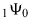and its incomplete variant formfor all r > 0, thereby simplifying and generalizing mainly the results (Nadarajah and Kotz 2007). The incomplete pψq is studied very recently by Srivastava and Pogány (2007). In what follows, the symbol pψq stands for the Fox-Wright generalization of the hypergeometric function pFq, with p numerator and q denominator parameters, defined byfor suitably bounded values of |x | and in terms of Euler's Gamma function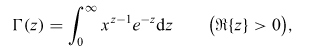where an empty product in (2) is to be interpreted (as usual) to be 1 (Mathai and Saxena 1978), (Srivastava et al. 1982). In terms of the analytically continued Gamma-function, the incomplete Gammafunction y (z, a) (Gradshteyn and Ryzhik 2000, 8.350 1.) is given by truncating the integration domain of Gamma-function to [0, ω], i.e.Following (Srivastava and Pogány 2007, Eq. (6)), we define the so-called incomplete Fox-Wright function pψq in the form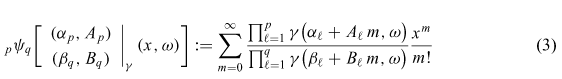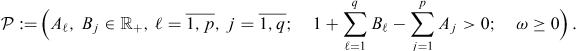It is interesting to remark, that the definition (3) immediately yields the limit relationship2 THE GAMMA-WEIBULL gW ( θ) DISTRIBUTION

Cutting off to (0, z) the integration domain+ of the integralintroduced by Nadarajah and Kotz (2007, Eq. (2.1)), we get the resulting integralsuch that it is closely connected to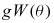distribution. We express nowin terms of the incomplete confluent FoxWrightfor positive real r+ extending the related result by Nadarajah and Kotz (2007, Eq. (2.2)), which was obtained for rational positive r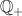.

LEMMA 1.PROOF. Expanding exp {  axr} into Maclaurin series, interchanging the order of the integration and summation and finally using definition of y(z; ω), then we easily confirm that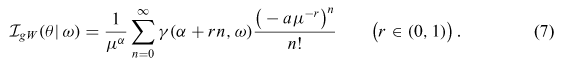which is the assertion of the Lemma. Since the constraint P in (3) being now ensured with r < 1, so the series (7) converges. The case r = 1 results in the incomplete gamma function. Finally, when r > 1, replacing xxr in the integral (5), we easily deduce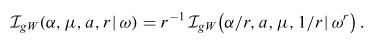This confirms the assertion of the Lemma. □

With the help of (4), we clearly deduce the limiting case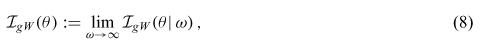and a fortiori the limiting results of Lemma 1. We, therefore, arrive at

COROLLARY 1.1.REMARK 1. The case r = 1 in (Nadarajah and Kotz 2007, Eq. (2.2)) is erroneous - there should be Γ(α) in the numerator.

THEOREM 1. Let the random variable, θ > 0 be with pdf (1). Then, the pdf fgW(x) and the PDF FgW (x) of r.v. ξ are of the form:respectively.

PROOF. To prove (10), we need the exact value of the normalizing constant K, such that appears in (1). But it is straightforward that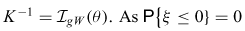hance, we deduce that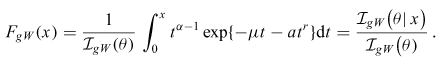Expanding the last relation into three formulse with respect to the related domains of r, by the Lemma 1 and Corollary 1.1 we conclude (11).

3 MOMENTS AND CARACTERISTIC FUNCTION

We now derive the momentsand the characteristic functionof the Gamma-Weibull distribution. All these results are expressed in terms of the confluent Fox-Wright functionvia the integralTHEOREM 2. The momentof order β of the r.v.is given by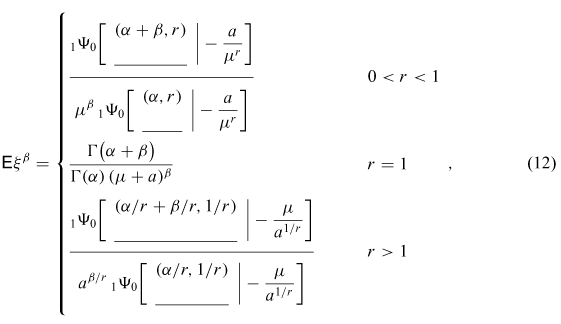wheneverMoreover, the associated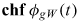is given byPROOF. Let us take a certain parameter β which satisfies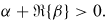Then, we have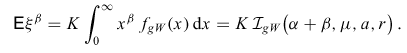Finally, for the chf, we have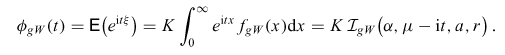Now, replacing, we immediately obtain (13).

4 CONCLUDING REMARKS

A. The confluent Fox-Wright functionsare defined by series containing gamma function and incomplete gamma function assumingwe have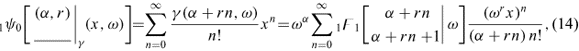because of (Srivastava et al. 1982, Eq. A25, p. 244)

Finally, applying now the limiting process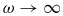to (14) and using (3), we deduceThe gamma-, incomplete gamma- and the confluent hypergeometricare implemented in Mathematica as the subroutinesand, respectively.

B. In practice, the parameter vectoris unknown, and it has to be estimated from a random sample X = (X1, ... , Xn), such that it consists of independently sampled identical random repliacse of the r.v.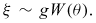The traditional parameter estimation procedure is the Maximum Likelihood (ML) method because the ML estimator possesses numerous desired properties, such as asymptotic normality and consistency. Unfortunately, this procedure can be used in a special case only for r = 1, whenbecomes the ordinary gamma-distribution with parameters α and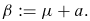It is known that the related ML-estimators are given bywhere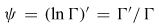stands for the familiar digamma function, anddenotes the sample mean.

In the case when r ≠ 1, in general the ML estimator does not exist. Indeed, let 0 < r < 1. Then, the log-likelihood function becomeswhereEquating to zero the partial derivatives of l0(X) with respect to μ and a, we conclude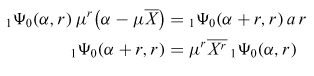such that it results in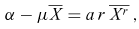whenNow, it is not difficult to find values of 0 for any fixed sample X outside of the manifold (15).

Similar procedure leads to counterexamples when r > 1.

Since the ML method is not efficient when r ≠ 1, following the recent method by Markovic et al. (2009) developed for the three-parameter Weibull distribution, Least Squares parameter estimation method could be derived for the gamma-Weibull distribution. However, this problem deserves a separate article.

ACKNOWLEDGMENTS

The authors are thankful to the referees for giving useful suggestions in the improvement of this article. The present investigation was partially supported by the Ministry of Sciences, Education and Sports of Croatia under Research Project No. 112-2352818-2814.

Manuscript received on June 25, 2008; accepted for publication on August 3, 2009

2010 Mathematics subject classification: Primary 33C90; Secondary 62E99.

• GRADSHTEYN IS AND RYZHIK IM. 2000. Table of Integrals, Series, and Products. (Corrected and Enlarged Edition prepared by A. Jeffrey and D. Zwillinger), 6th ed., Academic Press, New York.
• LEIPNIK RB AND PEARCE CEM. 2004. Independent non-identical five-parameter gamma-Weibull variates and their sums. ANZIAM J 46: 265-271.
• MARKOVIC D, JUKIC D AND BENSIC M. 2009. Nonlinear weighted leat squares estimation of a three-parameter Weibull density with a nonparametric start. J Comput Appl Math 228: 302-312.
• MATHAI AM AND SAXENA RK. 1978. The H-function with applications in statistics and other disciplines. Halsted Press, J Wiley & Sons, New York-London-Sidney.
• NADARAJAH S AND KOTZ S. 2007. On a distribution of Leipnik and Pearce. ANZIAM J 48: 405-407.
• SRIVASTAVA HM AND POGÁNY TK. 2007. Inequalities for a unified family of Voigt functions in several variables. Russ J Math Phys 14: 194-200.
• SRIVASTAVA HM, GUPTA KC AND GOYAL SP. 1982. The H-Functions of One and Two Variables with Applications. South Asian Publishers, New Delhi.
• Correspondence to:
Tibor K. Pogány
E-mail:

# Publication Dates

• Publication in this collection
11 June 2010
• Date of issue
June 2010